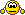The online racing simulator
Relation between RAF and Outsim Data
2
(35 posts, started )
Quote from Nitemare :...i just made a test, and measured length of forward and right vectors throughout a lap... and it stays constant : 37220

so they are unit vectors ... great one step further in understanding the data

Quote :so, how else could i get longitudial, lateral and vertical acceleration from RAF file???

...all there is in RAF is speed, distance, absolute coordinates, and orientation of the car...take the velocity vector project it along the cars coordinate system and build the time derivative (very easy since the raf output is discrete time)

edit:
then again atm i have no idea how to convert the scalar speed in the raf to a vector
Quote from Shotglass :take the velocity vector project it along the cars coordinate system and build the time derivative (very easy since the raf output is discrete time)

that is for longtitudial accelration... the tricky part is the lateral... we discussed it on czech forum and Chaos had an idea to use three points from cars trajectory (in times t-1, t and t+1) , make a triangle from them, and then make a circle that intersects all three vertexes... and then use radius of that circle in equation i wrote above...
no the idea is if you have a velocity vector (like you do using outsim data) in the xyz system you can poject that vector along the rfu (right forward up) axes of the cars system
then youll have longitudinal lateral and normal velocities which you can then derivate to work out the respective accel components
trouble is raf doesnt give you the velocity as a vector
Havent read the whole thread so I'm not sure if this is useful, but RAF gives you X, Y and Z position at 0.01s intervals so velocity vector is pretty easy to calculate for each timestep.
yup, but assuming that car is in turn and accelerating at the same time, there are two forces acting on it, one has the direction of velocity vector (tangent to the trajectory), and is a derivative of speed (let's call it aLON), the other one points into center of turn, and it is described by equation aLAT = v^2 / r
the force which i have to project to F and R vectors is then a = aLON + aLAT

anyway.. picture is worth a thousand words:#31 - w126
Car's accelaration is second time derivative of (X, Y, Z) vector. You can normalize right-vector and forward-vector (their length should be 1). You can also construct up-vector as their cross product. Then lateral accelaration is dot product of accelaration vector and normalized right-vector etc.
if youre driving around an ideal circle your scalar velocity wont change but the direction of the velocity will change a lot ... if you derive the velocity vectir youll get the cetripetal aceel as the radial component of the derivative in an (r \theta \phi) system
i dont understand.. how can i make a derivation of a vector?

(i suck at maths, sorry)
Can someone please provide me with some code to use to calculate pitch and roll from a RAF file?

That is, I'm looking for something similar to this from the RAF file documentation.

To work out heading from the forward-vector-------------------------------------------float b = FX / 32767.0f; // convert FX to a float from -1 to 1float e = FY / 32767.0f; // convert FY to a float from -1 to 1float heading = atan2(-b, e); // heading (anti-clockwise from above)

I haven't done this type of mathematics for years and it's doing my head in!
#35 - Juls
OMG, this is the Grail...I have been searching so long for this information:

" to work out a car's orientation matrix, the calculation order is : roll,

to work out the acceleration or velocity in the car's coordinate system, a
programmer should create horizontal (x) forward (y) and up (z) vectors
(using the heading, pitch and roll) and take the dot product (scalar
product) of these with the acceleration or velocity vectors supplied in the
outsim packet."

Maybe it could be somewhere in documentation (maybe it is?), because rotation order has to be known to properly convert world coordinates into car coordinates. I suppose most 3D gurus know about this order...but at least for me it was not so obvious (and angles come in the opposite order in OutSim packets!)
Here is the rotation matrix with this rotation order:
http://planning.cs.uiuc.edu/node102.html

edit: in the formula above you have to switch pitch and roll angles. Apply this matrix with alpha=LFS heading, beta=LFS roll, and gamma=LFS pitch and it seems to work fine.
2

Relation between RAF and Outsim Data
(35 posts, started )

© 2002-2020 Live for Speed - Scawen Roberts, Eric Bailey, Victor van Vlaardingen
All timestamps on this website are displayed in your local timezone
FGED GREDG RDFGDR GSFDG